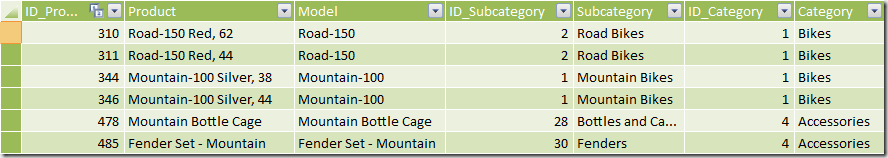The starting point is a PowerPivot workbook containing sales divided by Product, Date and Territory (it is an extraction of AdventureWorks sample database). The budget will be assigned to Product Category, Year and Month. Thus, you do not have the same granularity between Sales and Budget, because Territory is not present in budget and both Dates and Product tables are referenced at different granularities.

This is the Product table.This is the Dates table.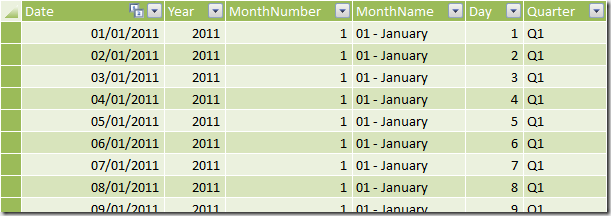This is the Territory table.And this is the Sales table.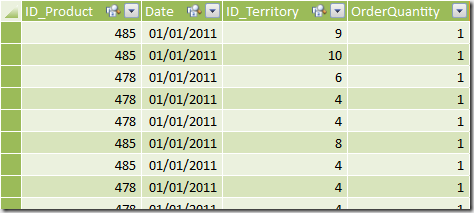As you can see, every row in the Sales table is related to a product to a single date, other than to a single territory. You can define the following relationships.After you created the required relationships, you can browse data in a PivotTable. For instance, you can see OrderQuantity value grouped by Product Category and Month in the following screenshot.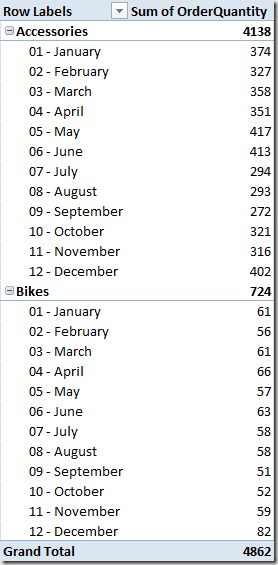In an Excel table you define the Budget table with Category, Year, Month and Budget columns (the Budget value will be compared to OrderQuantity).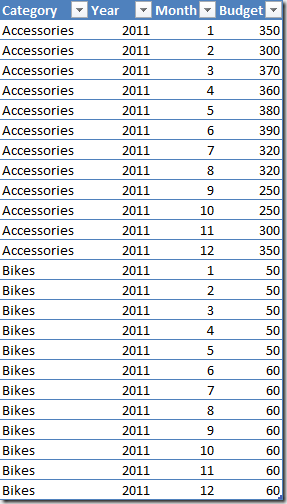At this point you cannot create a relationship between Budget and Dates or Product tables. The identity key in Dates table is the Date and in Product table is the Product, whereas you have a budget defined at an higher hierarchical level. But we do not have the notion of hierarchies in PowerPivot and BISM Tabular. An Analysis Services developer at this point might argue that a Multidimensional model can define relationships between fact and dimensions at different granularities, but this feature is not available here because the notion of attribute relationships inside a dimension simply doesn’t exist in Tabular. Thus, we have to rely on some DAX measure in order to make the comparison between OrderQuantity and Budget in a the same PivotTable, possibly without the need of modifying the data we already imported. In fact, a common solution is to create a table derived by Sales that group data by Product Category, Year and Month, resulting in a table that has the same granularity of the Budget one. However, this solution would require some form of ETL and is less flexible than the one proposed in this article.

If you consider the existing data, you might create a relationship between Budget[Category] and Product[Category] columns. This is not physically possible, but we will use this “virtual” relationship later in some DAX expression. A similar “virtual” relationship exists between Budget and Dates table, by using Year and Month columns. In order to to simplify the following DAX expression, it is better having a single column that allows a “virtual” relationship between these tables. You can create the YearMonth column in the Dates table by using the following DAX expression.

`=Dates[Year]*100+Dates[MonthNumber]`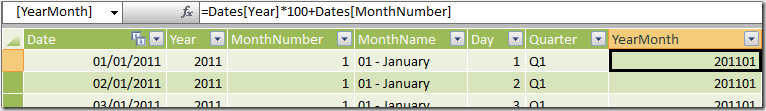And then you can create the YearMonth column in the Budget table in this way.

`=Budget[Year]*100+Budget[Month]`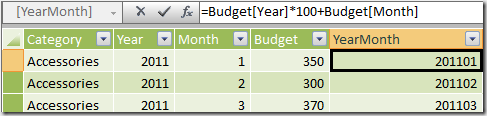Now you can write the BudgetCalc measure by using the following DAX expression.

```BudgetCalc :=
CALCULATE(
SUM( Budget[Budget] ),
FILTER( ALL( Budget[YearMonth] ),
COUNTROWS( FILTER( VALUES( Dates[YearMonth] ), Dates[YearMonth] = Budget[YearMonth] ) ) > 0 ),
FILTER( ALL( Budget[Category] ),
COUNTROWS( FILTER( VALUES( Product[category] ), Product[Category] = Budget[Category] ) ) > 0 )
)```

The CALCULATE statement calculates the sum of Budget values for all the corresponding rows in Budget table that have the same Year, Month and Product Category. Year and Month selection are handled by using the single YearMonth column, which simplify the writing of the required filter condition. In the new version of PowerPivot (SQL Server 2012) and in BISM Tabular, you can use a simpler syntax by using the new CONTAINS functions, such as in the following example.

```BudgetCalc :=
CALCULATE(
SUM( Budget[Budget] ),
FILTER( ALL( Budget[YearMonth] ),
CONTAINS( VALUES( Dates[YearMonth] ), Dates[YearMonth], Budget[YearMonth] ) ),
FILTER( ALL( Budget[Category] ),
CONTAINS( VALUES( Product[Category] ), Product[Category], Budget[Category] ) )
)
```

At this point you can add the BudgetCalc measure to the previous PivotTable obtaining a comparison between OrderQuantity and Budget values.However, you have some issue if you drill down data at the Subcategory level: the BudgetCalc is repeated for each Subcategory and this might misinterpreted by reading the result.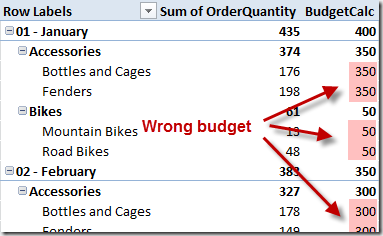The same problem arises if you try to drilldown data by any Territory attribute (such as Region, Country, or Group), and by Day or Date attributes of the Dates table.

In order to avoid this issue, it would be better to display a BLANK value for budget whenever you are navigating the PivotTable at a detail level that is not represented in the Budget table. In a Multidimensional model (formerly known as UDM in previous versions of Analysis Services), the IgnoreUnrelatedDimensions property helps you in obtaining this behavior. In order to do that in a Tabular model, you can create an IsBudgetValid measure that will return TRUE if the current selection in the PivotTable has a valid correspondent budget definition, FALSE otherwise.

```IsBudgetValid :=
(
COUNTROWS( Sales )
=
CALCULATE( COUNTROWS( Sales ),
ALL( Sales ),
VALUES( Dates[YearMonth] ),
VALUES( Product[Category] )
)
)

```

The IsBudgetValid measure checks that the number of rows that are active in the Sales table corresponds to the number of rows that would be active by removing from the filter context any column that is not part of the “virtual” relationship with the Budget table. Because we have just Category and YearMonth “virtual” relationships from Budget to Product and Dates table, the CALCULATE used to make the comparison replaces the filter context on Sales by removing the existing one using ALL and then inserting the filters on Category and YearMonth by using the VALUES function. In order to maintain this formula, you just have to insert a VALUES call for every column used as “virtual” relationship in the Budget table, without worrying about other changes in other part of the model.

As you can see in the following screenshot, the IsBudgetValid measure is FALSE for every row containing a wrong budget measure.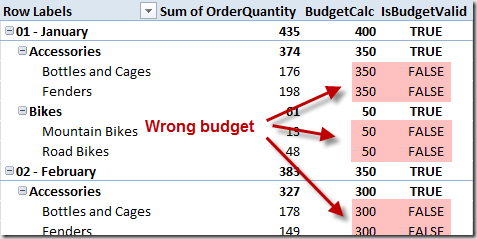As a side note, a much more complex alternative is to count the active rows for every dimension (every table but Sales), but this approach would require much more work to maintain the measure if other tables was added to the model.

```IsBudgetValidAlternative :=
(
COUNTROWS( Product )
= CALCULATE( COUNTROWS( Product ),
ALLEXCEPT( Product, Product[Category] ) )
) && (
COUNTROWS( Dates )
= CALCULATE( COUNTROWS( Dates ),
VALUES( Dates[YearMonth] ),
ALL( Dates ) )
) && (
COUNTROWS( Territory )
= COUNTROWS( ALL( Territory ) )
) ```

The IsBudgetValidAlternative measure checks that the number of rows that are active in every table of the PowerPivot workbook corresponds to the number of rows in the same table that corresponds to the granularity of the Budget table in the same filter context. For example, the number of rows in Product table is calculated in a CALCULATE function that alters the filter context in the Product table by using the ALLEXCEPT function, removing any filter other than the Category column, which is the only reference to the Product table from the Budget table. Such a number is compared with the number of rows active in Product obtained by calling a simple COUNTROWS. The same process has to be repeated for every table related to Sales, with a difference for Dates table caused by a particular handling of such table by PowerPivot engine. Finally, all unrelated tables should be tested by comparing the number of rows in the filter context with the number of rows in the whole table. As you can understand, this approach is much more expensive to write and to maintain.

Finally, you can create the Budget measure that shows the BudgetCalc value only when IsBudgetValid returns TRUE.

```Budget :=
IF( [IsBudgetValid], [BudgetCalc], BLANK() )```

The following screenshot show the Budget value browser by Month, Category and Model: as you can see, at the Model level the Budget is blank because the comparison cannot be made.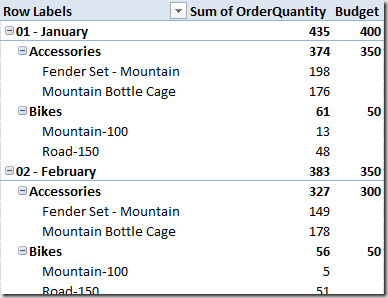In this article you have seen how to create DAX measures that compare data stored in tables at different granularities. You should use regular relationships in the PowerPivot or BISM Tabular model for the table with the higher granularity, and you can use DAX measures in order to display values from other tables that store data at an higher level of granularity. In the DAX expression you can define condition that produces the same effect of a “virtual” relationship defined using columns in a table that are not unique.

Acknowledgment: I want to thank Gerhard Brückl for inspiring me in writing this article.